### LeetCode-191 二进制位1的个数

LeetCode上第 191 号问题：编写一个函数，输入是一个无符号整数，返回其二进制表达式中数字位数为 ‘1’ 的个数。

``````class Solution:
def hammingWeight(self, n):
res = 0
while n != 0:
res += 1
n &= (n - 1)
return res

def hammingWeight2(self, n):
res = 0
while n != 0:
res += (n & 1)
n = n >> 1
return res
``````

### LeetCode-231 2的幂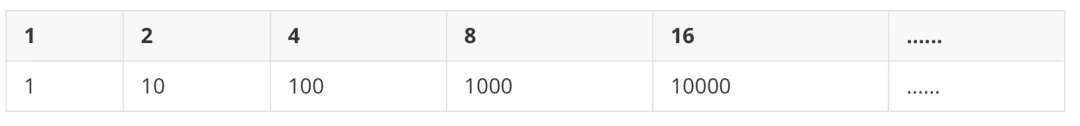``````def isPowerOfTwo(n):
res = 0
while n != 0:
res += (n & 1)
n >>= 1
return res == 1
``````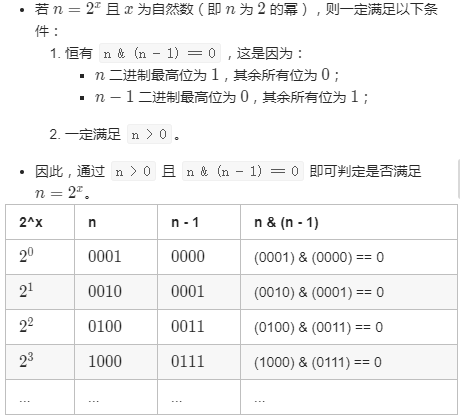``````class Solution:
def isPowerOfTwo(self, n: int) -> bool:
return (n > 0) and ((n & (n - 1)) == 0)
``````

### LeetCode-201. 闭区间范围内数字按位与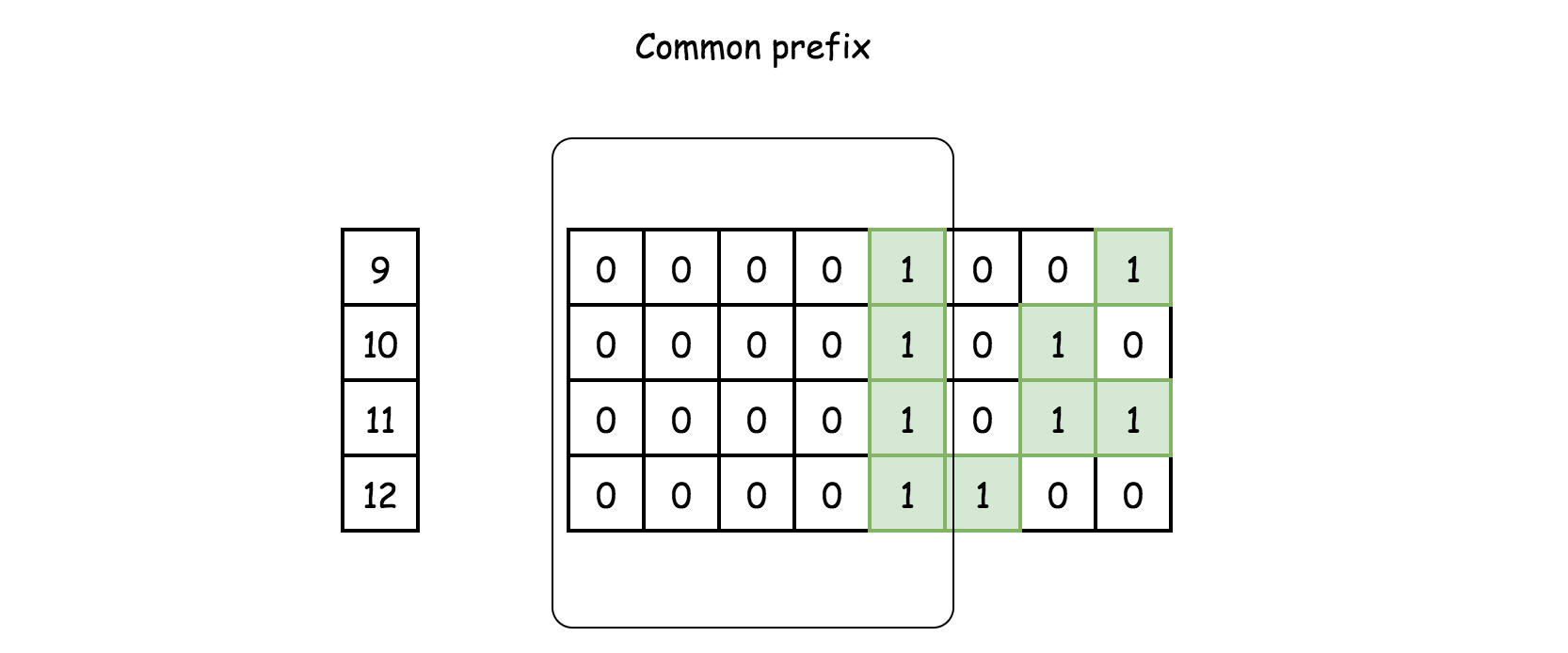1. 使用191的方法 `Brian Kernighan 算法` `n & (n-1) `
``````class Solution:
def rangeBitwiseAnd(self, m: int, n: int) -> int:
while m < n:
# turn off rightmost 1-bit
n = n & (n - 1)
return m & n
``````
1. 找m, n 的最高位1出现的位置 ， 如果不相等，则返回0，如果相等，则找公共前缀。B站视频-位运算练习【LeetCode】

### LeetCode-187.重复的DNA序列

``````输入：s = "AAAAACCCCCAAAAACCCCCCAAAAAGGGTTT"

``````
``````# 普通解法
class Solution:
def findRepeatedDnaSequences(self, s: str) -> List[str]:
d = {}
for i in range(len(s) - 9):
k = s[i: i+10]
if k in d:
d[k] = True
else:
d[k] = False

return [*filter(lambda x: d[x], d)]
``````

### LeetCode-36.只出现一次的数字

``````输入: [2,2,1]

``````

a b a⊕b
1 0 1
1 1 0
0 0 0
0 1 1
``````class Solution:
def singleNumber(self, nums: List[int]) -> int:
# 非空数组暂时不用判断
from functools import reduce
return reduce(lambda a, b: a ^ b, nums)
``````

### LeetCode-137.只出现一次的数字 II

``````输入: [2,2,3,2]

``````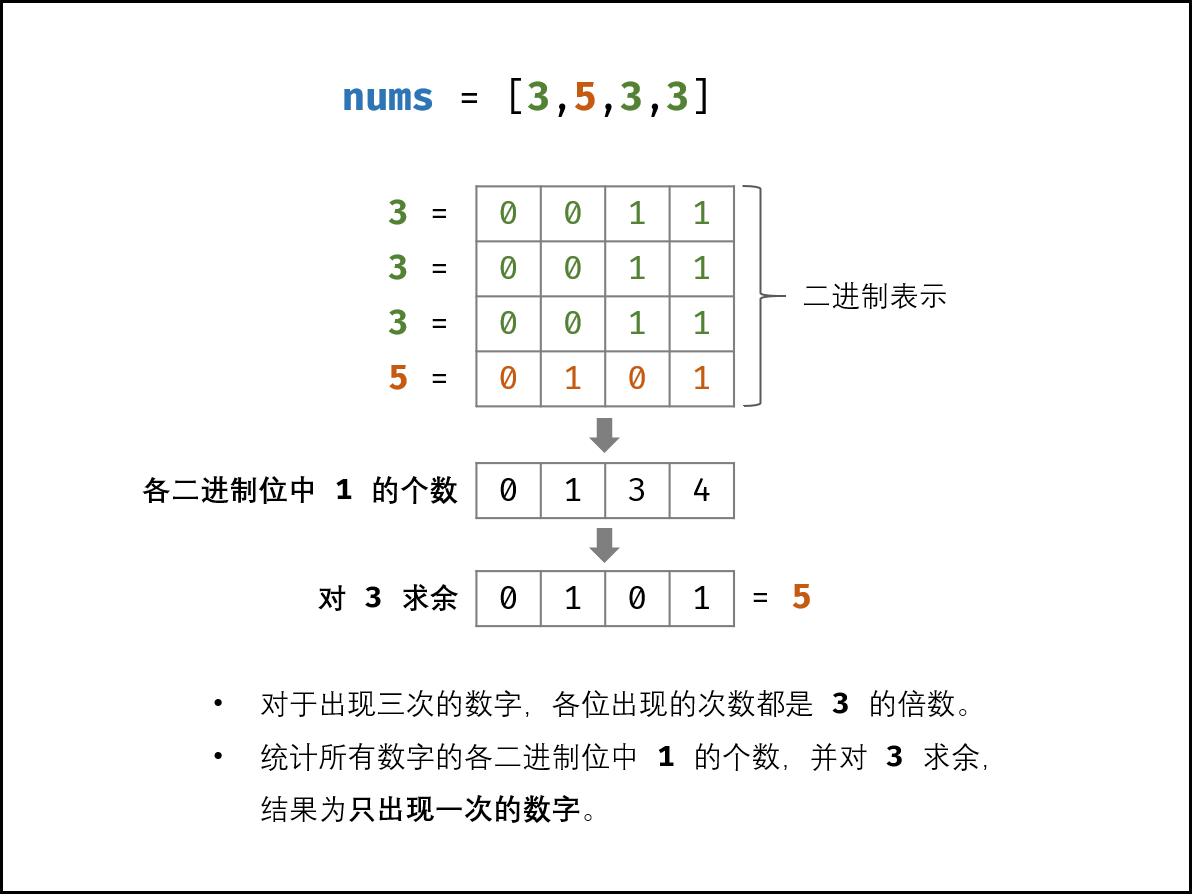3×(a+b+c)−(a+a+a+b+b+b+c)=2c 也可以应用在上一题

``````## 普通解法
class Solution:
def singleNumber(self, nums):
return (3 * sum(set(nums)) - sum(nums)) // 2
``````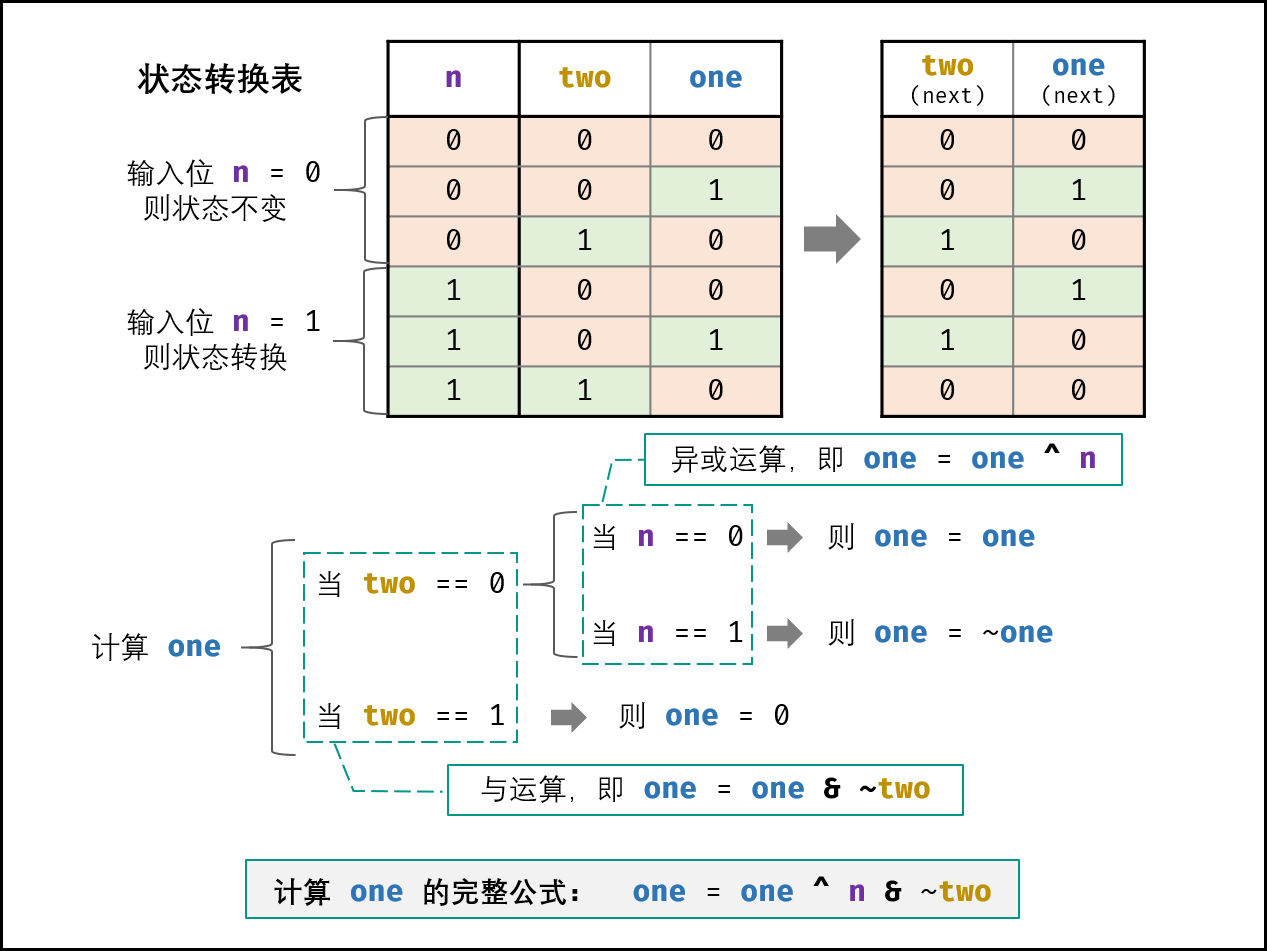``````class Solution:
def singleNumber(self, nums: List[int]) -> int:
ones, twos = 0, 0
for num in nums:
ones = ones ^ num & ~twos
twos = twos ^ num & ~ones
return ones
``````

### LeetCode-260. 只出现一次的数字 III

``````输入: [1,2,1,3,2,5]

``````

1. 结果输出的顺序并不重要，对于上面的例子， `[5, 3]` 也是正确答案。
2. 你的算法应该具有线性时间复杂度。你能否仅使用常数空间复杂度来实现？

``````class Solution:
def FindNumsAppearOnce(self, nums):
length = len(nums)
if length <= 0:
return nums
result = 0
# 先将所有数子异或得到一个值
for num in nums:
result ^= num
# 找到这个值最低位二进制位1的位置，根据这个位置来区分两个数组，分别异或求出只出现一次的数字
firstBitIndex = self.FindFirstBit(result)
n1, n2 = 0, 0
for num in nums:
if self.IsSameBit(num, firstBitIndex):
n1 ^= num
else:
n2 ^= num
return n1, n2

def FindFirstBit(self, num):
indexBit = 0
while num & 1 == 0:
indexBit += 1
num = num >> 1
return indexBit

def IsSameBit(self, num, indexBit):
num = num >> indexBit
return num & 1
``````
``````# 解放2
class Solution2:
def FindNumsAppearOnce(self, nums):
length = len(nums)
if length <= 0:
return []

diff = 0
for i in nums:
diff ^= i

n1, n2 = 0, 0
minDiff = self.getMinDiff(diff)
for num in nums:
if minDiff & num == 0:
n1 ^= num
n2 = diff ^ n1
return n1, n2

def getMinDiff(self, num):
# 保留一个低位是1的数字
# 取负号其实就是先取反，再加 1，需要 补码 的知识。最后再和原数相与就会保留最低位的 1。比如 1010，先取反是 0101，再加 1，就是 0110，再和 1010 相与，就是 0010 了
return num & (-num)
``````

### 如何得到二进制位只有一个1的数，几种方法

diff &= -diff ; 这里 的做法。

diff = (diff & (diff – 1)) ^ diff; 这里 的做法

`n & (n - 1)` 的操作在 191 题 用过，它可以将最低位的 1 置为 0。比如 `1110`，先将最低位的 1 置为 0 就变成 `1100`，然后再和原数 `1110 `异或，就得到了` 0010`

diff = xor & ~(diff – 1) 这里 的做法

``````mask=1